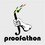# CMC - Problem 3

Problem 3. (3 points) In $$\triangle ABC$$, let $$D$$ be the midpoint of $$\overline{BC}$$. If $$AB=13$$, $$BC=14$$, and $$CA=15$$, find the maximum value of $$(A'B+A'D)(A'B-A'D)+(A'C+A'D)(A'C-A'D)$$, where $$A'$$ can be any point in the plane of $$\triangle ABC$$.Note by Cody Johnson
6 years, 8 months ago

This discussion board is a place to discuss our Daily Challenges and the math and science related to those challenges. Explanations are more than just a solution — they should explain the steps and thinking strategies that you used to obtain the solution. Comments should further the discussion of math and science.

When posting on Brilliant:

• Use the emojis to react to an explanation, whether you're congratulating a job well done , or just really confused .
• Ask specific questions about the challenge or the steps in somebody's explanation. Well-posed questions can add a lot to the discussion, but posting "I don't understand!" doesn't help anyone.
• Try to contribute something new to the discussion, whether it is an extension, generalization or other idea related to the challenge.

MarkdownAppears as
*italics* or _italics_ italics
**bold** or __bold__ bold
- bulleted- list
• bulleted
• list
1. numbered2. list
1. numbered
2. list
Note: you must add a full line of space before and after lists for them to show up correctly
paragraph 1paragraph 2

paragraph 1

paragraph 2

[example link](https://brilliant.org)example link
> This is a quote
This is a quote
    # I indented these lines
# 4 spaces, and now they show
# up as a code block.

print "hello world"
# I indented these lines
# 4 spaces, and now they show
# up as a code block.

print "hello world"
MathAppears as
Remember to wrap math in $$ ... $$ or $ ... $ to ensure proper formatting.
2 \times 3 $2 \times 3$
2^{34} $2^{34}$
a_{i-1} $a_{i-1}$
\frac{2}{3} $\frac{2}{3}$
\sqrt{2} $\sqrt{2}$
\sum_{i=1}^3 $\sum_{i=1}^3$
\sin \theta $\sin \theta$
\boxed{123} $\boxed{123}$

Sort by:

Is it 98?

- 6 years, 8 months ago

Yes, by the Median length theorem aka Apollonius' Theorem(you can call it a special case of Stewarts but the proof is trivial using cosine rule), the expression is equal to $A'B^2+A'C^2-2A'D^2=2BD^2=2*7^2=98$. This means that the expression stays invariant regardless of the location of $A'$.

More on the theorem: http://en.wikipedia.org/wiki/Apollonius'_theorem

Sometimes being well-known to these theorems and the structure of some equations can really help.

- 6 years, 8 months ago

I do in this way. First, I expand the given expression,

$(A'B + A'D)(A'B - A'D) + (A'C + A'D)(A'C - A'D) = (A'B)^{2} + (A'C)^{2} - 2(A'D)^{2} - (1)$

When we connect $A'B, A'D, A'C$, we will get two new triangles, i.e. $\Delta A'BD$ and $\Delta A'CD$. So, I apply The Theorem of Stewart,

$7(A'B)^{2} + 7(A'C)^{2} = 14((A'D)^{2} +49)$

Divide 7 on both sides,

$(A'B)^{2} + (A'C)^{2} = 2((A'D)^{2} + 49)$

Expand RHS and subtract $2(A'D)^{2}$ from both sides,

$(A'B)^{2} + (A'C)^{2} - 2(A'D)^{2} = 98$

Comparing the equation above with $(1)$, we get

$(A'B + A'D)(A'B - A'D) + (A'C + A'D)(A'C - A'D) = \boxed {98}$

- 6 years, 8 months ago

Correct! 3 points for you!

- 6 years, 8 months ago

Thanks!

- 6 years, 8 months ago

Too sleepy to solve it right now, but a little piece of humble advice on the problem-writing. The way you have defined $A'$ is not logically incorrect but it is a bit confusing and it is not the standard way of writing it. Here is how I would rephrase the problem. Also, "in" is not completely rigorous.

Let $ABC$ be a triangle such that $AB = 13$, $BC = 14$, and $CA = 15$. Let $D$ be the midpoint of $\overline {BC}$. Let $A'$ be a point coplanar to $\triangle ABC$. Find the maximum possible value of $\left( A'B + A'D \right) \cdot \left(A'B - A'D\right) + \left(A'C + A'D\right) \cdot \left(A'C - A'D\right).$

Hope you don't take this as criticism or egotistical advice; rather, as suggestions on problem-writing.

- 6 years, 8 months ago

I had that at first but was tentative. Thanks for your criticism, I genuinely appreciate it.

- 6 years, 8 months ago

You're welcome. Also, tags? ;)

- 6 years, 8 months ago